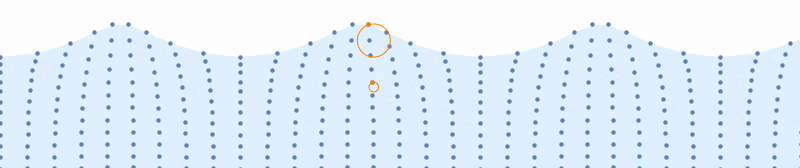# Animating wave motion in water

Further to this question I found on MSE, I tried to replicatefrom here

this is as far as I got:

fun[a_, b_, c_, x_, y_] :=
Point[{#[] + x, #[] + y} &[
Part[CirclePoints c,
If[a + b == 360, 360, Mod[a + b, 360]]]]];
tab = With[{a = #},
Flatten[Table[
Table[fun[a, 90 + 15 n, 1 - .15 m, -1 + .5 n, -.35 m], {m, 0,
10}], {n, 0, 24}], 1]] & /@ Range[1, 360, 15];

Module[{t, x, y, fun, xf, yf, a}, x = -.5; y = 1;
fun[a_, b_, c_, x_, y_] :=
Point[{#[] + x, #[] + y} &[
Part[CirclePoints c,
If[a + b == 360, 360, Mod[a + b, 360]]]]];
xf[t_, a_, b_] := a t - b Sin[t]; yf[t_, a_, b_] := a - b Cos[t];
Animate[
Show[
Graphics[
{PointSize[.01], tab[[a]]},
PlotRange -> {{-1 - x, 10 + x}, {-1 - y, 1}}
],
ParametricPlot[
{(Pi/2) xf[t + 2 Pi a/24, 1.25, .6] - 4 Pi a/24 - Pi^2 + .05,
2.05 - 1.65 yf[t + 2 Pi a/24, 1.25, .6]},
{t, -4 Pi, 4 Pi}, Axes -> False
]
],
{a, 1, 24, 1}, ControlPlacement -> Top, AnimationRate -> 5,
AnimationDirection -> Backward
]
]


which is not very efficient (I'm sure Part could be applied more efficiently), and despite various tweeks, I couldn't quite manage to get the cycloid to line up with the points.

What is a better way to approach this?

• – LCarvalho Mar 18 '19 at 11:23
• For searching purposes: this is a trochoidal wave. – J. M.'s technical difficulties Mar 22 '19 at 7:07
• @J.M.isslightlypensive thanks - looks like next step will be stokes drift! – martin Mar 22 '19 at 8:01

DynamicModule[{t = 0, d = 5, a = .08, base, distortion, pts, r, f, n = 10},

r[y_] := .08 y^4;
f[x_] := -2 Pi Dynamic[t] + d x;
(*f does not evaluate to a number but FE will take care of that later*)

base = Array[List, n {3, 1}, {{0, Pi}, {0, 1}} ];

distortion = Array[
Function[{x, y}, r[y] {Cos @ f @ x, Sin @ f @ x}], n {3, 1}, {{0, Pi}, {0, 1}}
];

pts = base + distortion;

Row[{
Animator[Dynamic @ t, AnimationRate -> .8, AppearanceElements -> {}],
Graphics[{
LightBlue,
Polygon @ Join[ pts[[;; , -1]], {Scaled[{1, 0}], Scaled[{0, 0}]}],

Darker @ Blue, AbsolutePointSize @ 5, Point @ Catenate @ pts,

AbsolutePointSize @ 7, Orange, Thick,
Point @ pts[[15, -1]],  Circle[base[[15, -1]], r @ base[[15, -1, 2]]],
Point @ pts[[15, 7]],  Circle[base[[15, 7]], r @ base[[15, 7, 2]]]
},
PlotRange -> {{0 + .1, Pi - .1}, {0, 1.2}},
PlotRangeClipping -> True, ImageSize -> 800]
}]
]• thanks - a vastly better approach! – martin Mar 18 '19 at 13:34
• @martin thanks, let me know if anything is not clear. – Kuba Mar 19 '19 at 21:31
• a = .08 doesn't get used; maybe you meant r[y_] := a y^4? (Also, how did you pick the form of r[y]?) – J. M.'s technical difficulties Mar 22 '19 at 14:50
• @J.M.isslightlypensive right, should be deleted. About formulas, I got them from looking at the original .gif :-) I was too lazy to think about physics etc so I assumed something like that should work. I also ignored OP's code :( – Kuba Mar 22 '19 at 14:55

This is, as J.M. pointed out, a trochoidal wave. I'm going to provide an implementation based on this. This is slightly different compared to what Kuba did. The advantage is that this parametrization makes it easy to decide the wavelength, wave height, propagation speed and more. It even lets you account for gravity to get realistic waves (trochoidal waves are actual solutions to the Euler equations of fluid motion).

Wikipedia provides formulae for the position $$(X, Y)$$ for each of the dots in the visualization given the center of the corresponding circle $$(a, b)$$ and time $$t$$.

$$X(a, b, t) = a + \frac{e^{kb}}{k}\sin(k(a+ct))$$ $$Y(a, b, t) = b - \frac{e^{kb}}{k}\cos(k(a+ct))$$

Note the constants $$k$$ and $$c$$, which determine the wavelength and the speed of propagation. We can also determine from these variables the radius of the corresponding circle.

The implementation is as follows:

\[Lambda] = 3;
k = 2 \[Pi]/\[Lambda];
c = 9.82/k;
x[a_, b_, t_] := a + Exp[k b]/k Sin[k (a + c t)]
y[a_, b_, t_] := b - Exp[k b]/k Cos[k (a + c t)]
r[{a_, b_}] := Exp[k b]/k

xmin = 0.;
xmax = 10.;
ymin = -2.;
ymax = -0.4;
nx = 30;
ny = 11;
coords = CoordinateBoundsArray[
{{xmin, xmax}, {ymin, ymax}},
{(xmax - xmin)/nx, (ymax - ymin)/ny}
];

surface[t_] := {x[#, #2, t], y[#, #2, t]} & @@@ Part[coords, All, -1];
all[t_] := {x[#, #2, t], y[#, #2, t]} & @@@ Flatten[coords, 1];
selected1 = coords[[15, -1]];
selected2 = coords[[15, -5]];

plot[t_] := Graphics[{
LightBlue,
Polygon[Join[surface[t], {{xmax, ymin}, {xmin, ymin}}]],
ColorData[97, 1],
PointSize[Medium],
Point[all[t]],
Orange,
Circle[selected1, r[selected1]],
Circle[selected2, r[selected2]],
Point[{x[#, #2, t], y[#, #2, t]} & @@ selected1],
Point[{x[#, #2, t], y[#, #2, t]} & @@ selected2]
},
PlotRange -> {{xmin, xmax}, {ymin, ymax + 0.5}},
ImageSize -> 800
]

Manipulate[plot[t], {t, 0, 10}]• +1 Very helpful visualization to learn about waves as a sailor. The parameterization should help a lot to play with it in a “realistic” fashion. – gwr May 5 '19 at 7:57